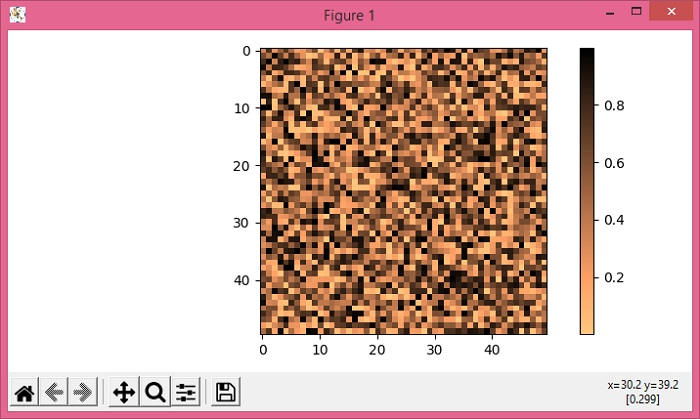# How to plot a 2D matrix in Python with colorbar Matplotlib?

To plot a 2D matrix in Python with colorbar, we can use numpy to create a 2D array matrix and use that matrix in the imshow() method.

## Steps

• Create data2D using numpy.

• Use imshow() method to display data as an image, i.e., on a 2D regular raster.

• Create a colorbar for a ScalarMappable instance *mappable* using colorbar() method and imshow() scalar mappable image.

• To display the figure, use show() method.

## Example

import numpy as np
from matplotlib import pyplot as plt
plt.rcParams["figure.figsize"] = [7.00, 3.50]
plt.rcParams["figure.autolayout"] = True
data2D = np.random.random((50, 50))
im = plt.imshow(data2D, cmap="copper_r")
plt.colorbar(im)
plt.show()

## OutputUpdated on: 15-May-2021

5K+ Views